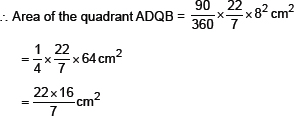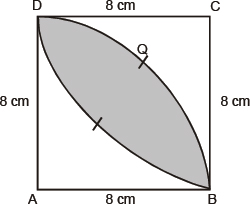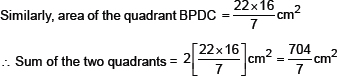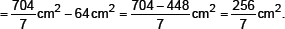# Areas related to Cirles-NCERT Solutions

Class X Math
NCERT Solution for Areas Related to Circles
Q.1.   The radii of two circles are 19 cm and 9 cm respectively. Find the radius of the circle which has circumference equal to the sum of the circumferences of the two circles.
Sol. We have, r1 = 19 cm
r2 = 9 cm
∴ Circumference of circle-I = 2π r1 = 2π (19) cm
Circumference of circle-II = 2π r1 = 2π (19) cm
Sum of the circumferences of circle-I and circle-II
= 2π (19) + 2π (9) = 2π (19 + 9) cm = 2π (28) cm
Let R be the radius of the circle-III.
∴ Circumference of circle-III = 2π R
According to the condition,
2π R = 2π (28)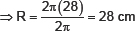Thus, the radius of the new circle = 28 cm.
Q.2.   The radii of two circles are 8 cm and 6 cm respectively. Find the radius of the circle having area equal to the sum of the areas of the two circles.
Sol. We have,
Radius of circle-I, r1 = 8 cm
∴ Area of circle-I = πr1 2 = π(8)2 cm2
Area of circle-II = r22 = π(6)2 cm2
Let the area of the circle-III be R
∴ Area of circle-III = πr2
Now, according to the condition,
πr12 + πr22 = πr2
i.e. π(8)2 + π(6)2 = πr2
⇒ π(82 + 62) = πr2
⇒ 82 + 62 = r2
⇒ 64 + 36 = r2
⇒ 100 = r2
⇒ 102 = r2 ⇒ R = 10
Thus, the radius of the new circle = 10 cm.
Q.3.   Figure depicts an archery target marked with its five scoring regions from the centre outwards as Gold, Red, Blue, Black and White. The diameter of the region representing gold score is 21 cm and each of the other bands is 10.5 cm wide. Find the area of each of the five scoring regions.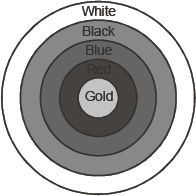Sol. Diameter of the innermost region = 21 cm
Radius of the innermost (Gold Scoring) region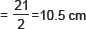∴ Area of Gold region = π (10.5)2 cm2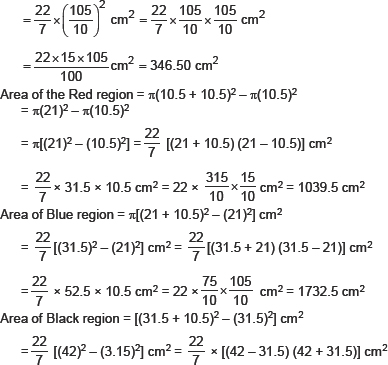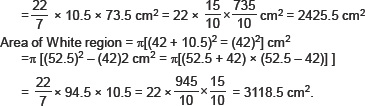Q.4.   The wheels of a car are of diameter 80 cm each. How many complete revolutions does each wheel make in 10 minutes when the car is travelling at a speed of 66 km per hour?
Sol. Diameter of a wheel = 80 cm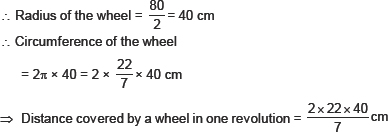Distance travelled by the car in 1hr
= 66 km = 66 × 1000 × 100 cm
∴ Distance travelled in 10 minutes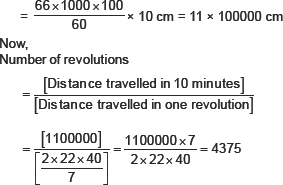Thus, the required number of revolutions = 4375.
Q.5.   Tick the correct answer in the following and justify your choice: If the perimeter and the area of a circle are numerically equal, then the radius of the circle is
(A) 2 units
(B) π units
(C) 4 unites
(D) 7 units
Sol. We have:
[Numerical area of the circle] = [Numerical circumference of the circle]
⇒ πr2 = 2π r
⇒ πr2 – 2π r = 0
⇒ r2 – 2 r = 0
⇒ r(r – 2) = 0
⇒ r = 0 or r = 2
But r cannot be zero
∴ r = 2 units.
Thus, the option (A) 2 units is correct.
•   Area of Sector and Segment of a Circle
The portion (or part) of the circular region enclosed by two radii and the corresponding are is called a sector of the circle.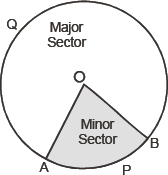The portion (or part) of the circular region enclosed between a chord and the corresponding arc is called a segment of the circle.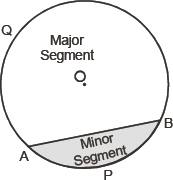Exercise 12.2
Q.1.   Find the area of a sector of a circle with radius 6 cm if angle of the sector is 60°.
Sol. Here, r = 6 cm
θ = 60°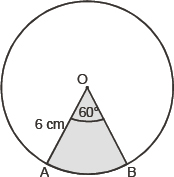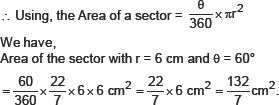Q.2.   Find the area of a quadrant of a circle whose circumference is 22 cm.
Sol. Let radius of the circle = r
∴ 2πr = 22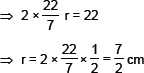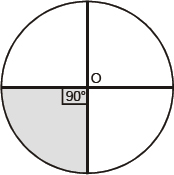∴ Area of the quadrant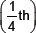of the circle,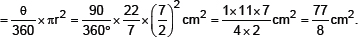Q.3.   The length of the minute hand of a clock is 14 cm. Find the area swept by the minute hand in 5 minutes.
Sol. [Length of minute hand] = [radius of the circle]
⇒ r = 14 cm
θ Angle swept by the minute hand in 60 minutes = 360°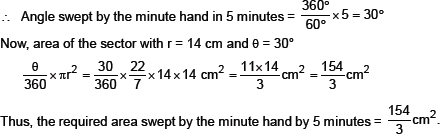Q.4.   A chord of a circle of radius 10 cm subtends a right angle at the centre. Find the area of the corresponding (i) minor segment (ii) major sector.
Sol. Length of the radius (r) = 10 cm
Sector angle θ = 90°
Area of the sector with θ = 90° and r = 10 cm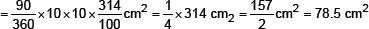Now,
(i) Area of the minor segment
= [Area of minor sector] – [Area of rt. ΔAOB]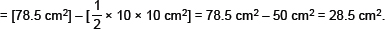(ii) Area of major segment
= [Area of the circle] – [Area of the minor segment]
= πr2 – 78.5 cm2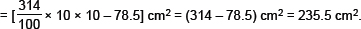Q.5.   In a circle of radius 21 cm, an arc subtends an angle of 60° at the centre. Find:
(i) the length of the arc
(ii) area of the sector formed by the arc
(iii) area of the segment formed by the corresponding chord
Sol. Here, radius = 21 cm and θ = 60°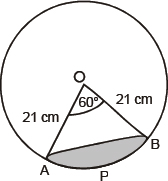(i) Circumference of the circle = 2 πr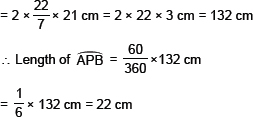(ii) Area of the sector with sector angle 60°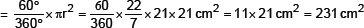(iii) Area of the segment APQ
= [Area of the sector AOB] – [Area of ΔAOB]                                                ...(1)
In ΔAOB, OA = OB = 21 cm
∴∠A = ∠B = 60°                                                                                                      [θ∠O = 60°]
⇒AOB is an equilateral Δ,
∴AB = 21 cm
Draw OM ⊥ AB such that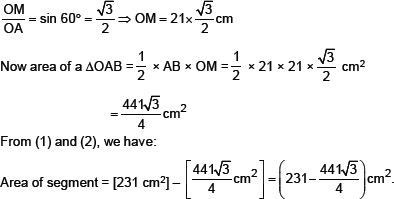Q.6.   A chord of a circle of radius 15 cm subtends an angle of 60° at the centre. Find the areas of the corresponding minor and major segments of the circle.
Sol. Here, radius (r) = 15 cm
Sector angle θ = 60°
∴ Area of the sector with θ = 60°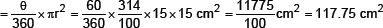Since ∠O = 60° and OA = OB = 15 cm
∠AOB is an equilateral triangle.
⇒ AB = 15 cm and ∠A = 60°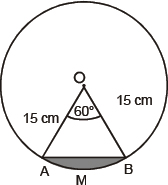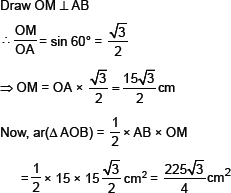Now area of the minor segment
= (Area of minor sector) – (ar ΔAOB)
= (117.75 – 97.3125) cm2 = 20.4375 cm2
Area of the major segment
= [Area of the circle] – [Area of the minor segment]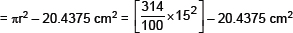= 706.5 – 20.4375 cm2 = 686.0625 cm2.
Q.7.   A chord of a circle of radius 12 cm subtends an angle of 120° at the centre. Find the area of the corresponding segment of the circle.
Sol. Here, θ = 120° and r = 12 cm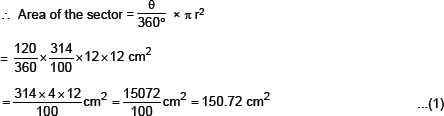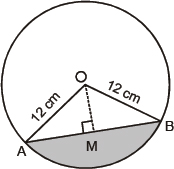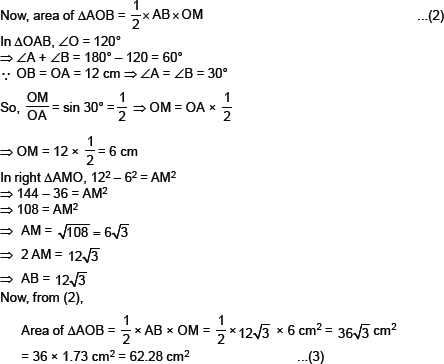From (1) and (3)
Area of the minor segment
= [Area of minor segment] – [Area of ΔAOB]
= [150.72 cm2] – [62.28 cm2] = 88.44 cm2.
Q.8.   A horse is tied to a peg at one corner of a square shaped grass field of side 15 m by means of a 5m long rope (see figure). Find:
(i) the area of that part of the field in which the horse can graze.
(ii) the increase in the grazing area if the rope were 10m long instead of 5m.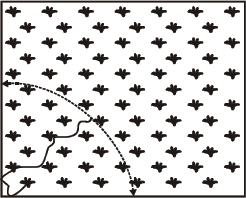Sol. Here, Length of the rope = 5 m
∴ Radius of the circular region grazed by the horse = 5 m
(i) Area of the circular portion grazed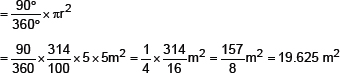(ii) When length of the rope is increased to 10 m,
∴ r = 10 m
⇒ Area of the circular region where θ = 90°.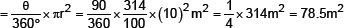∴ Increase in the grazing area
= 78.5 – 19.625 m2 = 58.875 m2.
Q.9.   A brooch is made with silver wire in the form of a circle with diameter 35 mm. The wire is also used in making 5 diameters which divide the circle into 10 equal sectors as shown in Fig. Find:
(i) the total length of the silver wire required.
(ii) the area of each sector of the brooch.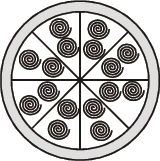Sol. Diameter of the circle = 35 mm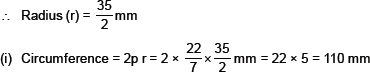Length of 1 piece of wire used to make diameter to divide the circle into 10 equal sectors = 35 mm
∴ Length of 5 pieces = 5 × 35 = 175 mm
∴ Total length of the silver wire = 110 + 175 mm = 285 mm
(ii) Since the circle is divided into 10 equal sectors,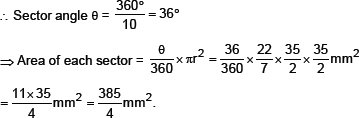Q.10.   An umbrella has 8 ribs which are equally spaced (see figure).
Assuning umbrella to be a flat circle of radius 45 cm, find the area between the two consecutive ribs of the umbrella.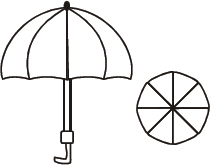Sol. Here, radius (r) = 45 cm
Since circle is divided in 8 equal parts,
∴ Sector angle corresponding to each part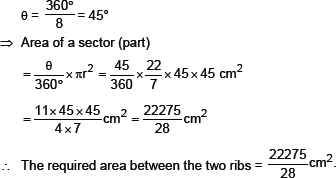Q.11.   A car has two wipers which do not overlap. Each wiper has a blade of length 25 cm sweeping through an angle of 115°. Find the total area cleaned at each sweep of the blades.
Sol. Here, radius (r) = 25 cm
Sector angle (θ) = 115°
∴ Area cleaned by each sweep of the blades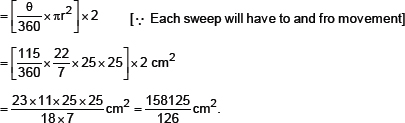Q.12.   To warn ships for underwater rocks, a lighthouse spreads a red coloured light over a sector of angle 80° to a distance of 16.5 km. Find the area of the sea over which the ships are warned.
Sol. Here, Radius (r) = 16.5 km
Sector angle (θ) = 80°
∴ Area of the sea surface over which the ships are warned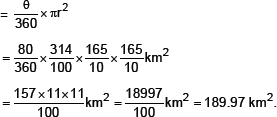Q.13.   A round table cover has six equal designs as shown in Fig. If the radius of the cover is 28 cm, find the cost of makign the designs at the rate of Rs. 0.35 per cm2.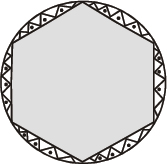Sol. Here, r = 28 cm
Since, the circle is divided into six equal sectors.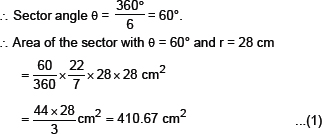Now, area of 1 design
= Area of segment APB
= Area of sector – Area of ΔAOB                                                                                                                ...(2)
In ΔAOB, ∠AOB = 60°, OA = PN = 28 cm
∴ ∠OAB = 60° and ∠OBA = 60°
⇒ ΔAOB is an equilateral triangle.
⇒ AB = AO = BO
⇒ AB = 28 cm
Draw OM ⊥AB
∴ In right ΔAOM, we have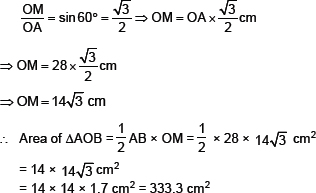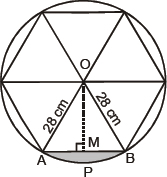Now, from (1), (2) and (3), we have:
Area of segment APQ = 410.67 cm2 – 333.2 cm2 = 77.47 cm2
⇒ Area of 1 design = 77.47 cm2
∴ Area of the 6 equal designs = 6 × (77.47) cm2
= 464.82 cm2
Cost of making the design at the rate of Rs. 0.35 per cm2,
= Rs. 0.35 × 464.82
= Rs. 162.68.
Q.14.   Tick the correct answer in the following:
Area of a sector of angle p (in degrees) of a circle with radius R is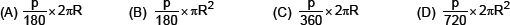Sol. Here, radius (r) = R
Angle of sector (θ) = p°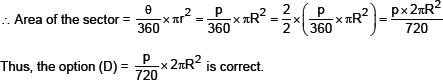Exercise 12.3
Q.1.   Find the area of the shaded region in Fig. PQ = 24 cm, PR = 7 cm and O is the centre fo the circle.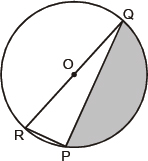Sol. Since O is the centre of the circle,
∴ QOR is a diameter.
⇒ ∠RPQ = 90°                                                                        [Angle in a semi-circle]
Now, in right DRPQ,
RQ2 = PQ2 + PR2
⇒ RQ2 = 242 + 72 = 756 + 49 = 625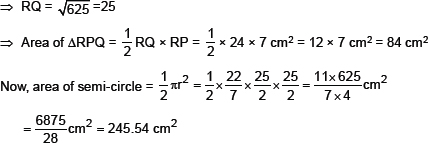∴ Area of the shaded portion = 245.54 cm2 – 84 cm2 = 161.54 cm2.
Q.2.   Find the area of the shaded region in figures, if radii of the two concentric circles with centre O are 7 cm and 14 cm respectively and ∠AOC = 40°.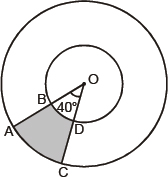Sol. Radius of the outer circle = 14 cm
Here, θ = 40°
∴ Area of the sector BOD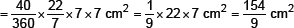Now, area of the shaded region
= [Area of sector AOC] – [Area of sector BOD]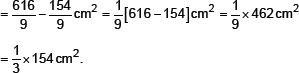Q.3.   Find the area of the shaded region in figure, if ABCD is a square of side 14 cm and APD and BPC are semi-circles.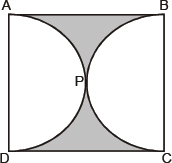Sol. Side of the square = 14 cm
∴ Area of the square ABCD = 14 × 14 cm2 = 196 cm2
Now, diameter of the circle = (Side of the square) = 14 cm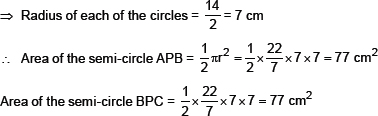= [Area of the square] – [Area of semi-circle APD + Area of semi-circle BPC]
= 196 – [77 + 77] cm2 = 196 – 154 cm2 = 42 cm2.
Q.4.   Find the area of the shaded region in figure, where a circular arc of radius 6 cm has been drawn with vertex O of an equilateral triangle OAB of side 12 cm as centre.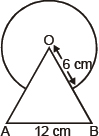Sol. Area of the circle with radius = 6 cm.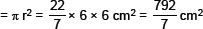Area of equilateral triangle, having side a = 12 cm, is given by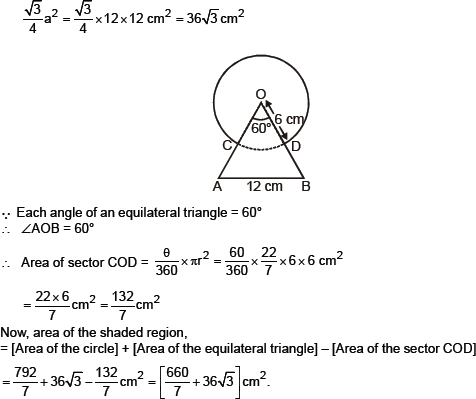Q.5.   From each corner of a square of side 4 cm a quadrant of a circle of radius 1 cm is cut and also a circle of diameter 2 cm is cut as shown in figure. Find the area of the remaining portion of the square.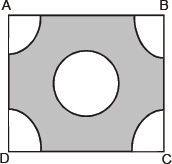Sol. Side of the square = 4 cm
∴ Area of the square ABCD = 4 × 4 cm2 = 16 cm2
∴ Area of all the 4 quadrant squares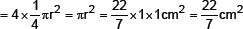Diameter of the middle circle = 2 cm
⇒ Radius fo the middle circle = 1 cm
∴ Area of the middle circle = πr2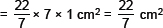Now, area of the shaded region
= [Area pf tje sqiare ABCD] – [(Area of the 4 quadrant circles) + (Area of the middle circle)]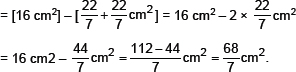Q.6.   In a circular table cover of radius 32 cm, a design is formed leaving an equilateral triangle ABC in the middle as shown in figure. Find the area of the design.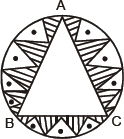Sol. Area of the circle having radius r = 32 cm.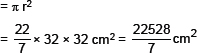‘O’ is the centre of the circle,
∴ AO = OB = OC = 32 cm
⇒ ∠AOB = ∠BOC = ∠AOC = 120°
Now, in ΔAOB, ∠1 = 30°
∵ ∠1 + ∠2 = 60°
Also OA = OB ⇒ ∠1 = ∠2
If OM ⊥AB, then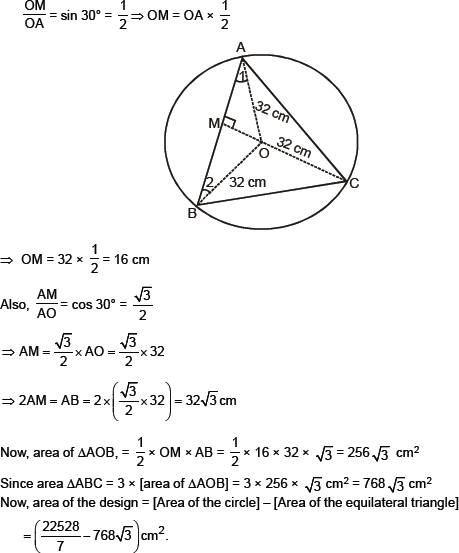Q.7.   In figure, ABCD is a square of side 14 cm. With centres A, B, C and D, four circles are drawn such that each circle touch externally two of the remainign three circles. Find the area of the shaded region.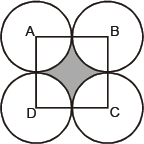Sol. Side of the square ABCD = 14 cm
∴ Area of the square ABCD = 14 × 14 cm2 = 196 cm2.
θ Circles touch each other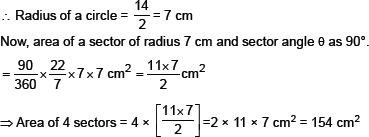∴ Area of the shaded region = [Area of the square ABCD] – [Area of the 4 sectors]
= 196 cm2 – 154 cm2 = 42 cm2.
Q.8.   The figure depicts a racing track whose left and right ends are semicircular.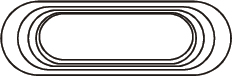The distance between the two inner parallel line segments is 60 m and they are each 106 m long.
If the track is 10 m wide, find:
(i) the distance around the track along its inner edge
(ii) the area of the track.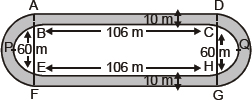Sol. (i) Distance around the track along its inner edge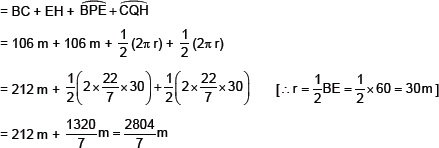(ii) Now, area of the track
= Area of the shaded region
= (Area of rectangle ABCD) + (Area of rectangle EFGH)
+ 2 [(Area of 2 semi-circles each of radius 40 m) – (Area of 2 sem-circles each of radius 30 cm)]
⇒ Area of the track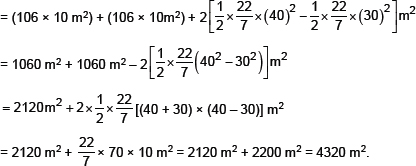Q.9.   In the figure, AB and CD are two diameters of a circle (with centre O) perpendicular to each other and OD is the diameter of the smaller circle. If OA = 7 cm, find the area of the shaded region.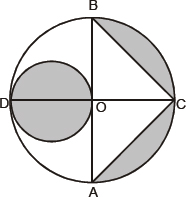Sol. O is the centre of the circle.
OA = 7 cm
⇒ AB = 2 OA = 2 × 7 = 14 cm
OC = OA = 7 cm
θ AB and CD are perpendicular to each other
⇒ OC ⊥ AB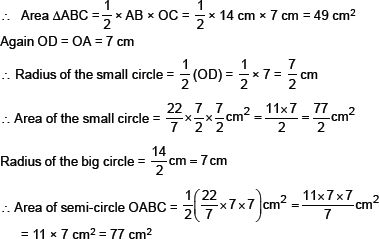Now, Area of the shaded region
= [Area of the small circle] + [Area of the big semi-circle OABC] – [Area of ΔABC]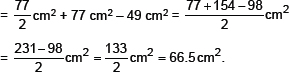Q.10.   The area of an equilateral triangle ABC is 17320.5 cm2. With each vertex of the triangle as centre, a circle is drawn with radius equal to half the length of the side of the triangle (see Fig.). Find the area of the shaded region.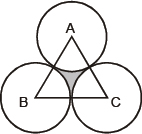Sol. Area of ΔABC = 17320.5 cm2
∵ΔABC is an equilateral triangle and area of an equilateral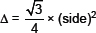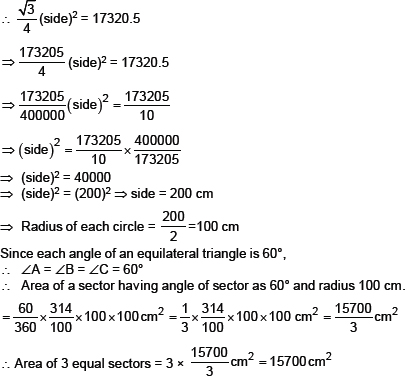Now, area of the shaded regino
= [Area of the equilateral triangle ABC] – [Area of 3 equal sectors]
= 17320.5 cm2 – 15700 cm2 = 1620.5 cm2.
Q.11.   On a square handkerchief, nine circular designs each of radius 7 cm are made (see figure). Find the area of the remaining portion of the handkerchief.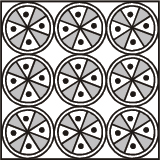Sol. θ The circles touch each other.
∴ The side of the square ABCD
= 3 × diameter of a circle
= 3 × (2 × radius of a circle) = 3 × (2 × 7 cm)
= 42 cm
⇒ Area of the square ABCD = 42 × 42 cm2 = 1764 cm2.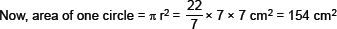∵ There are 9 squares
∴ Total area of 9 circles = 154 × 9 = 1386 cm2
∴ Area of the remaining portion of the handkerchief = 1764 – 1386 cm2 = 378 cm2.
Q.12.   In the figure, OACB is a quadrant of a circle with centre O and radius 3.5 cm. If OD = 2 cm, find the area of the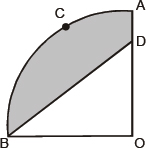Sol. Here, centre of the circle is O and radius = 3.5 cm.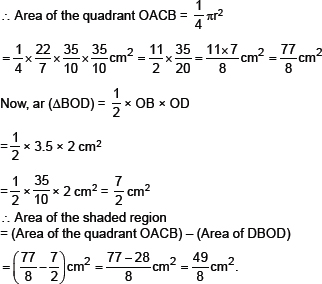Q.13.   In the figure, a square OABC is inscribed in a quadrant OPBQ. If OA = 20 cm, find the area of the shaded region.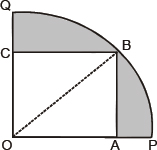Sol. OABC is a square such that its side OA = 20 cm.
∴ OB2 = OA2 + OB2
= [202 + 202] = [400 + 400] =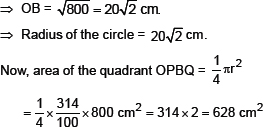Area of the square OABC = 20 × 20 cm2 = 400 cm2
∴ Area of the shaded region = 268 cm2 – 400 cm2 = 228 cm2.
Q.14.   AB and CD are respectively areas of two concentric circles of radii 21 cm and 7 cm and centre O (see figure). If ∠AOB = 30°, find the area of the shaded region.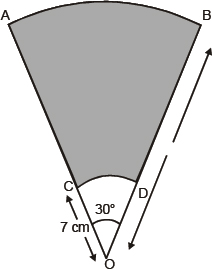R = 21 cm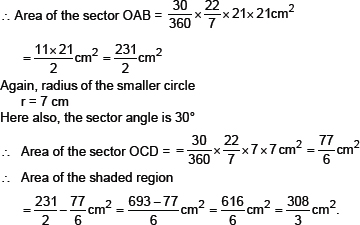Q.15.   In the figure, ABPC is a quadrant of a circle of radius 14 cm and a semi-circle is drawn with BC as diameter. Find the area of the shaded region.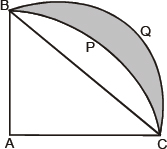Therefore, area of the quadrant ABPC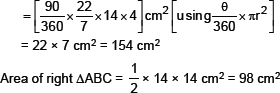⇒ Area of segment BPC = 154 cm2 – 98 cm2 = 56 cm2
Now, in right ΔABC,
AC2 + AB2 = BC2
⇒ 142 + 142 = BC2
⇒ 196 + 196 = BC2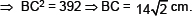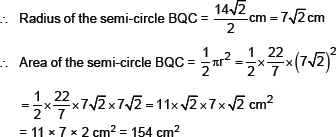Now, area of the shaded region
= [Area of segment BQC] – [Area of segment BPC]
= 154 cm2 – 56 cm2 = 98 cm2.
Q.16.   Calculate the area of the designed region in the figure, common between the two quadrants of circles of radius 8 cm each.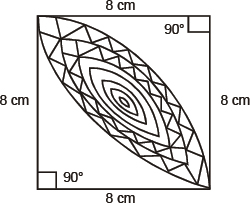Sol. θ Side of the square = 8 cm
∴ Area of the square (ABCD) = 8 × 8 cm2
= 64 cm2